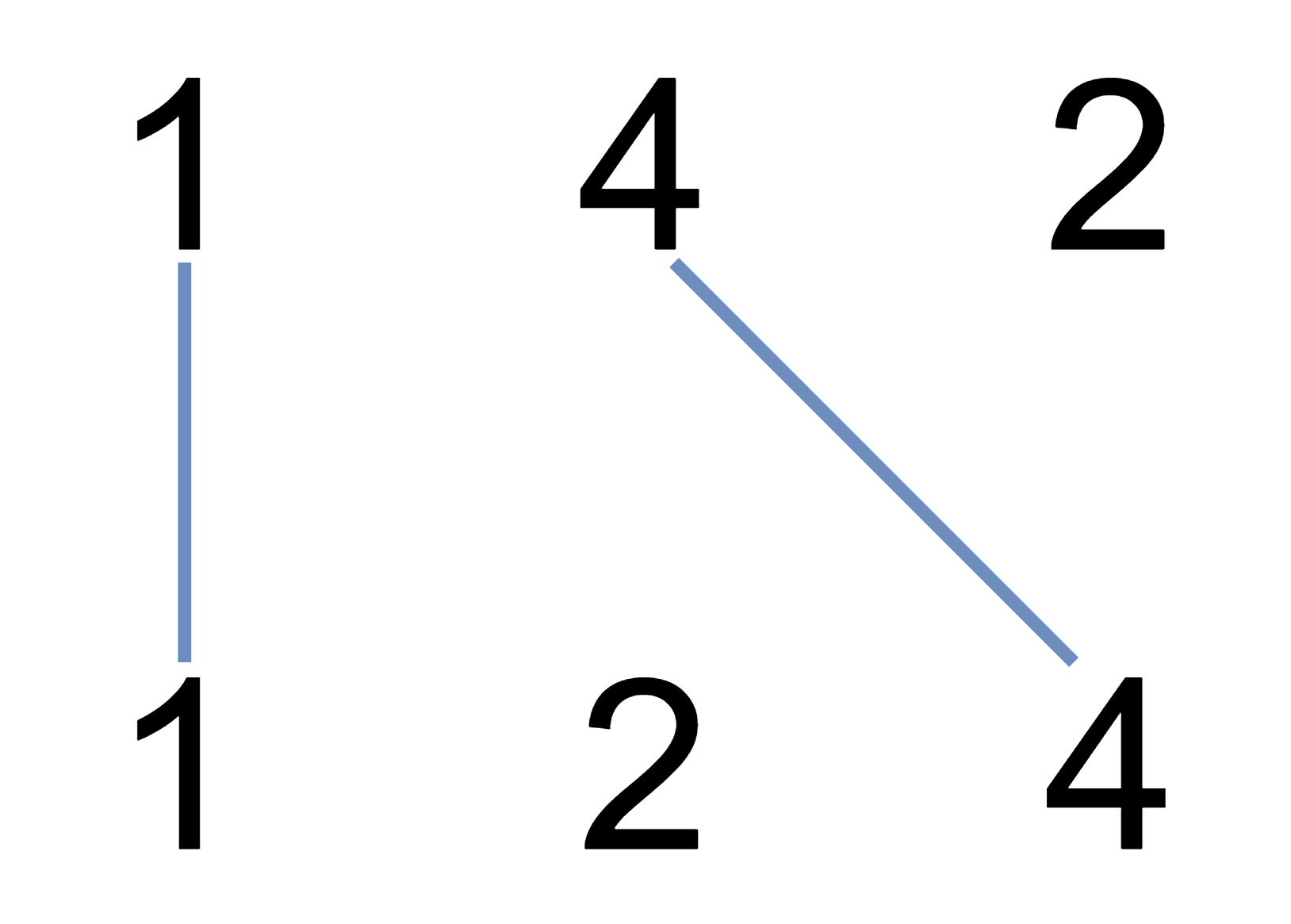1035. Uncrossed Lines

We write the integers of `A` and `B` (in the order they are given) on two separate horizontal lines.

Now, we may draw connecting lines: a straight line connecting two numbers `A[i]` and `B[j]` such that:

• `A[i] == B[j]`;
• The line we draw does not intersect any other connecting (non-horizontal) line.

Note that a connecting lines cannot intersect even at the endpoints: each number can only belong to one connecting line.

Return the maximum number of connecting lines we can draw in this way.

Example 1:```Input: A = [1,4,2], B = [1,2,4]
Output: 2
Explanation: We can draw 2 uncrossed lines as in the diagram.
We cannot draw 3 uncrossed lines, because the line from A=4 to B=4 will intersect the line from A=2 to B=2.
```

Example 2:

```Input: A = [2,5,1,2,5], B = [10,5,2,1,5,2]
Output: 3
```

Example 3:

```Input: A = [1,3,7,1,7,5], B = [1,9,2,5,1]
Output: 2```

Note:

1. `1 <= A.length <= 500`
2. `1 <= B.length <= 500`
3. `1 <= A[i], B[i] <= 2000`

1035. Uncrossed Lines
``````struct Solution;

impl Solution {
fn max_uncrossed_lines(a: Vec<i32>, b: Vec<i32>) -> i32 {
let n = a.len();
let m = b.len();
let mut dp = vec![vec![0; m + 1]; n + 1];
for i in 0..n {
for j in 0..m {
if a[i] == b[j] {
dp[i + 1][j + 1] = dp[i][j] + 1;
} else {
dp[i + 1][j + 1] = dp[i][j + 1].max(dp[i + 1][j]);
}
}
}
dp[n][m]
}
}

#[test]
fn test() {
let a = vec![1, 4, 2];
let b = vec![1, 2, 4];
let res = 2;
assert_eq!(Solution::max_uncrossed_lines(a, b), res);
let a = vec![2, 5, 1, 2, 5];
let b = vec![10, 5, 2, 1, 5, 2];
let res = 3;
assert_eq!(Solution::max_uncrossed_lines(a, b), res);
let a = vec![1, 3, 7, 1, 7, 5];
let b = vec![1, 9, 2, 5, 1];
let res = 2;
assert_eq!(Solution::max_uncrossed_lines(a, b), res);
}
``````